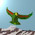## Wednesday, January 18, 2012

### Witten on Yang Mills

Witten speaks at the latest Simons Center conference on mass gaps in Yang Mills theories. In four dimensions, he stresses that the role of the mass gap is to hide quantum phenomena at large distances, and that no simple perturbation theory can really see the mass gap. A theme of the conference seems to be a renewal of interest in constructive approaches, starting with tractable questions.

So the talk focuses on three dimensional theories, starting with the pure Yang Mills action for $R^3$. Here a mass gap exists on dimensional grounds, with $m = w g^2$ for $w$ some constant and $g$ the coupling. But what happens at long distances in three dimensions? Several approaches are briefly reviewed, including (i) a string tension Hamiltonian approach, and (ii) Feynman and Singer's Hamiltonian for the space $R^2$. In (ii) there is the usual (infinite dimensional) space of connections $A$ and gauge transformations $G$. Witten writes down the basic Hamiltonian

$H = g^2 \int \textrm{Tr} E^2 + \frac{1}{g^2} \int \textrm{Tr} B^2$

noting that the first term dominates in the limit $g^2 \rightarrow \infty$, and that the eigenvalues of $H$ should be basic numbers, like $\zeta (2)$.

But he appears to believe that a currently tractable approach to mass gaps would look at non-trivial CFTs, for the following reasons. First observe that (in three dimensions) if we add a few essentially massless fermions (like up and down quarks) there is no mass gap. Now we can add a Chern-Simons term, as a mass gap term, to the usual Yang-Mills action, where $m$ is proportional to $k$, the CS integral coefficient (the fourth dimension is irrelevant). If this (CS) theory is rigorously defined in three dimensions, there should be a theory for any $3$-manifold $M$ with any Riemannian metric. Scale the metric by a factor $t \rightarrow \infty$. That is, we imagine that human experiments are limited by the characteristic scale of the manifold $M$, and at long distances there is the TFT (IR limit). This is of course the context of Jones polynomials and knots.

#### 1 comment:

1.Jaffe discusses constructive approaches. Unfortunately, by this he means only the local ones. I gave up at slide $5$.

Note: Only a member of this blog may post a comment.